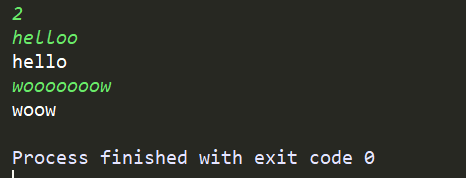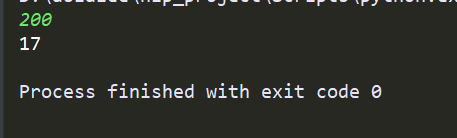# 字符串自动校对——2019字节跳动笔试题目

### 字符串自动校对（修正拼写错误字符串）

helloo

wooooooow

woow

#字符串修正函数
def correction_string(s):
n = len(s)
r = list(s[:min(len(s), 2)])
for i in range(2, n):
if s[i] == r[-1] == r[-2]:
continue
if s[i] == r[-1] and len(r) > 2 and r[-2] == r[-3]:
continue
r.append(s[i])
new_string = ''.join(r)
return new_string

for i in range(int(input())):
s=input()
print(correction_string(s))题目描述：Z国的货币系统包含面值1元、4元、16元、64元共计四种硬币，以及面值1024元的纸币。现在小Y使用1024元的纸币购买了一件价值为N的商品，请问最少他会收到多少硬币。

输入格式：共一行，包含整数N。

输出格式：共一行，包含一个数，表示最少收到的硬币数。

数据范围：0<N≤1024

输入样例：200

输出样例：17

样例解释：花200，需要找零824块，找12个64元硬币，3个16元硬币，2个4元硬币即可。

surplus_coin=1024-int(input())
number=0
coin_list=[64,16,4,1]
for i in coin_list:
number+=surplus_coin//i
surplus_coin=surplus_coin%i
print(number)07-1122504-11722
12-111033
08-142万+
05-191531